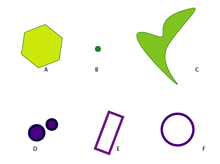# Contractible spaceIllustration of some contractible and non-contractible spaces. Spaces A, B, and C are contractible; spaces D, E, and F are not.

In mathematics, a topological space X is contractible if the identity map on X is null-homotopic, i.e. if it is homotopic to some constant map. Intuitively, a contractible space is one that can be continuously shrunk to a point within that space.

## Properties

A contractible space is precisely one with the homotopy type of a point. It follows that all the homotopy groups of a contractible space are trivial. Therefore any space with a nontrivial homotopy group cannot be contractible. Similarly, since singular homology is a homotopy invariant, the reduced homology groups of a contractible space are all trivial.

For a topological space X the following are all equivalent (here Y is an arbitrary topological space):

• X is contractible (i.e. the identity map is null-homotopic).
• X is homotopy equivalent to a one-point space.
• X deformation retracts onto a point. (However, there exist contractible spaces which do not strongly deformation retract to a point.)
• Any two maps f,g: YX are homotopic.
• Any map f: YX is null-homotopic.

The cone on a space X is always contractible. Therefore any space can be embedded in a contractible one (which also illustrates that subspaces of contractible spaces need not be contractible).

Furthermore, X is contractible if and only if there exists a retraction from the cone of X to X.

Every contractible space is path connected and simply connected. Moreover, since all the higher homotopy groups vanish, every contractible space is n-connected for all n ≥ 0.

## Locally contractible spaces

A topological space is locally contractible if every point has a local base of contractible neighborhoods. Contractible spaces are not necessarily locally contractible nor vice versa. For example, the comb space is contractible but not locally contractible (if it were, it would be locally connected which it is not). Locally contractible spaces are locally n-connected for all n ≥ 0. In particular, they are locally simply connected, locally path connected, and locally connected.

## References

1. Munkres, James R. (2000). Topology (2nd ed.). Prentice Hall. ISBN 0-13-181629-2.
2. Hatcher, Allen (2002). Algebraic Topology. Cambridge University Press. ISBN 0-521-79540-0.